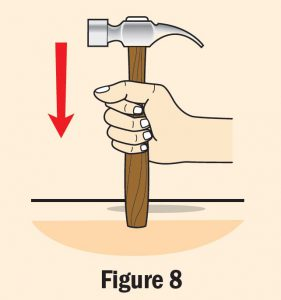# Summative Practice (Question 8 & 9) – Form 4 Science (KSSM) Chapter 11

Question 8:Figure 7 shows a ticker tape chart of a trolley moving down an inclined runway. The ticker timer being used vibrates at a frequency of 50 Hz.

(a) Determine the time for 10 ticks.

(b) What is the velocity for ticker tape 1 and ticker tape 5?

(c) The runway is inclined further so that the trolley moves down faster. Predict the length of the ticker tape chart that will be obtained.

(a) 0.02 s × 10 = 0.2 s

(b)
Ticker tape 1:
10 cm ÷ 0.2 s = 50 cm s–1

Ticker tape 5:
50 cm ÷ 0.2 s = 250 cm s–1

(c)
The length of each ticker tape strip will become longer because the distance between ticks increases.

Question 9:Figure 8 shows a student banging the handle of a hammer against a hard surface.

(a) What is the actual purpose of the student doing so?

(b) What is the concept used by the student?

(c) Explain two of your daily activities that use the concept stated in question 9(b).